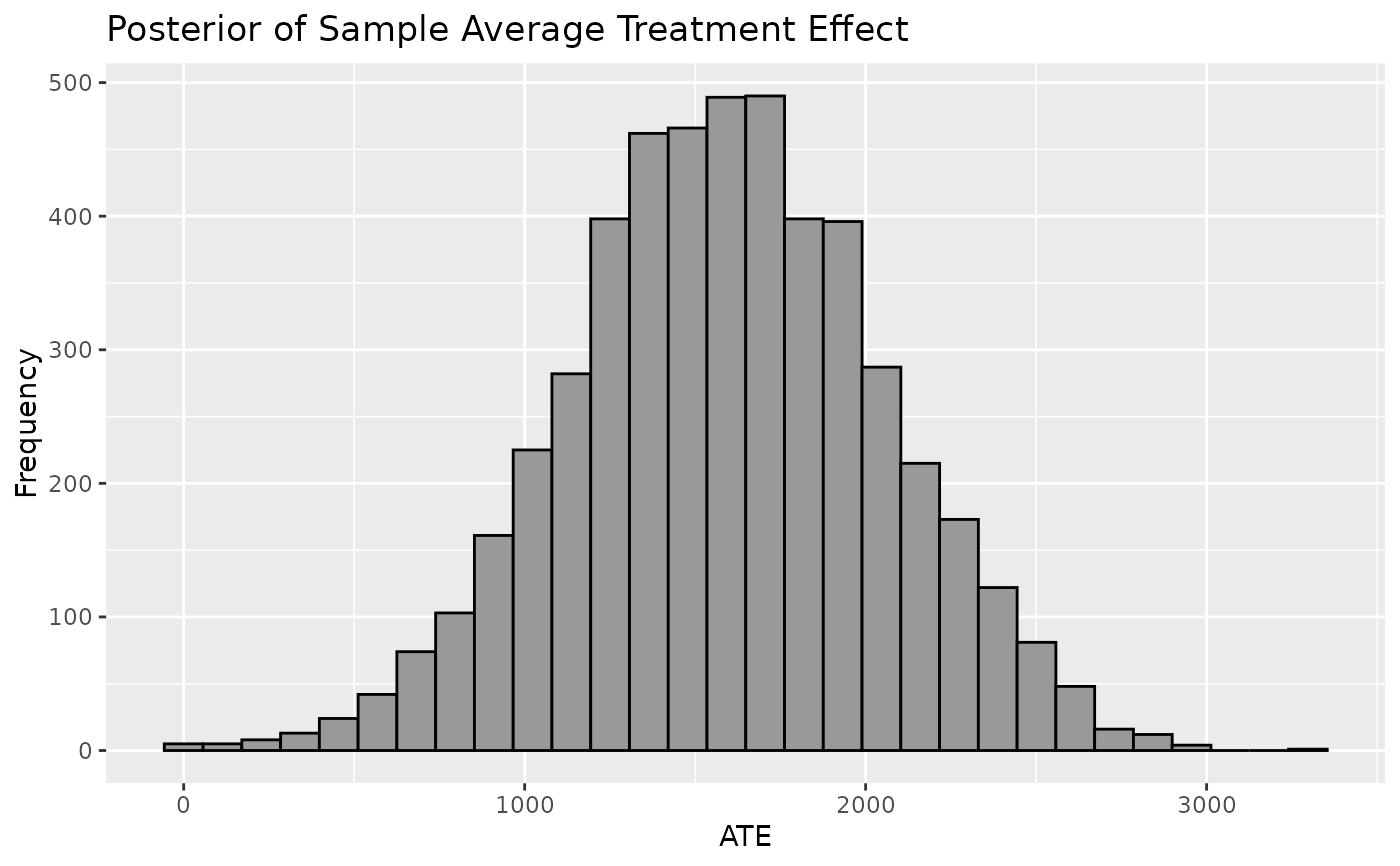Plot shows the Population Average Treatment Effect which is derived from the posterior predictive distribution of the difference between $$y | z=1, X$$ and $$y | z=0, X$$. Mean of PATE will resemble CATE and SATE but PATE will account for more uncertainty and is recommended for informing inferences on the average treatment effect.

## Usage

plot_PATE(
.model,
type = c("histogram", "density"),
ci_80 = FALSE,
ci_95 = FALSE,
reference = NULL,
.mean = FALSE,
.median = FALSE
)

## Arguments

.model

a model produced by bartCause::bartc()

type

histogram or density

ci_80

TRUE/FALSE. Show the 80% credible interval?

ci_95

TRUE/FALSE. Show the 95% credible interval?

reference

numeric. Show a vertical reference line at this value

.mean

TRUE/FALSE. Show the mean reference line

.median

TRUE/FALSE. Show the median reference line

ggplot object

## Author

George Perrett, Joseph Marlo

## Examples

# \donttest{
data(lalonde)
confounders <- c('age', 'educ', 'black', 'hisp', 'married', 'nodegr')
model_results <- bartCause::bartc(
response = lalonde[['re78']],
treatment = lalonde[['treat']],
confounders = as.matrix(lalonde[, confounders]),
estimand = 'ate',
commonSup.rule = 'none'
)
#> fitting treatment model via method 'bart'
#> fitting response model via method 'bart'
plot_PATE(model_results)
#> stat_bin() using bins = 30. Pick better value with binwidth.# }